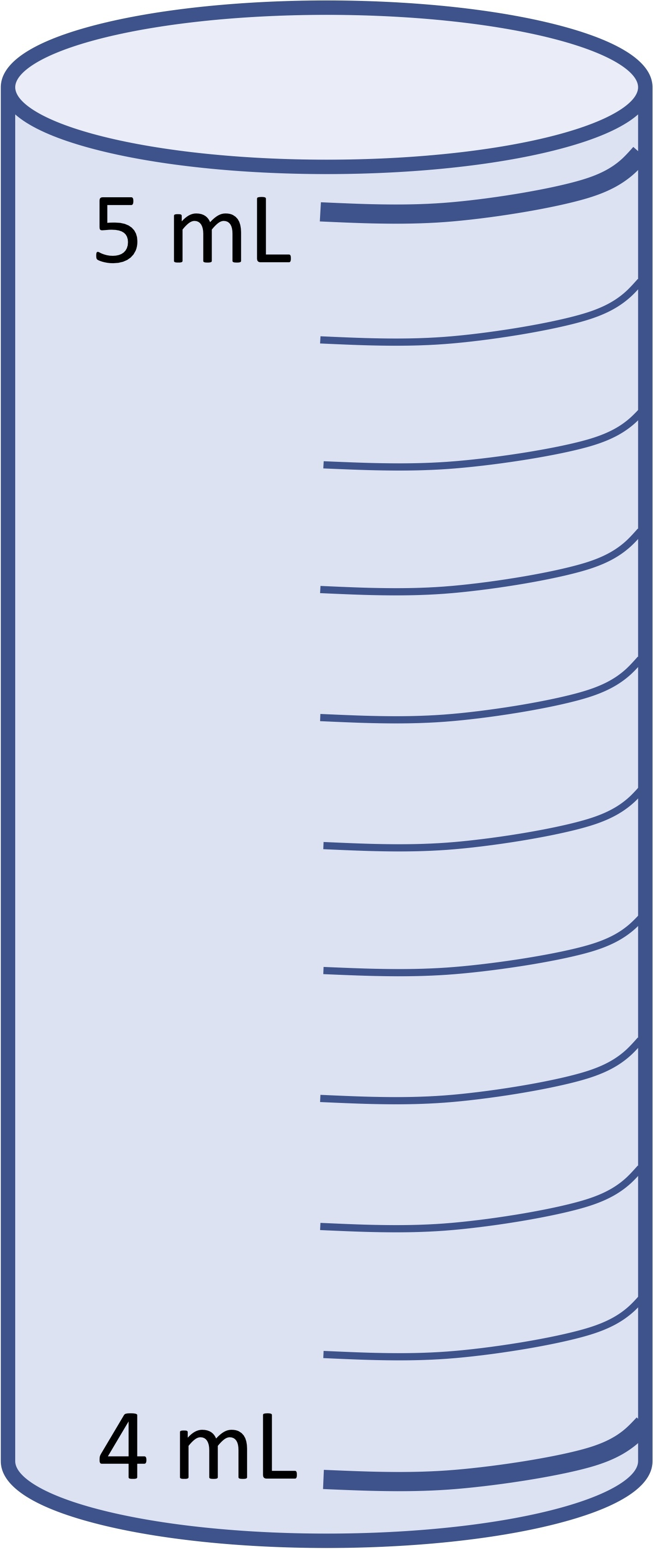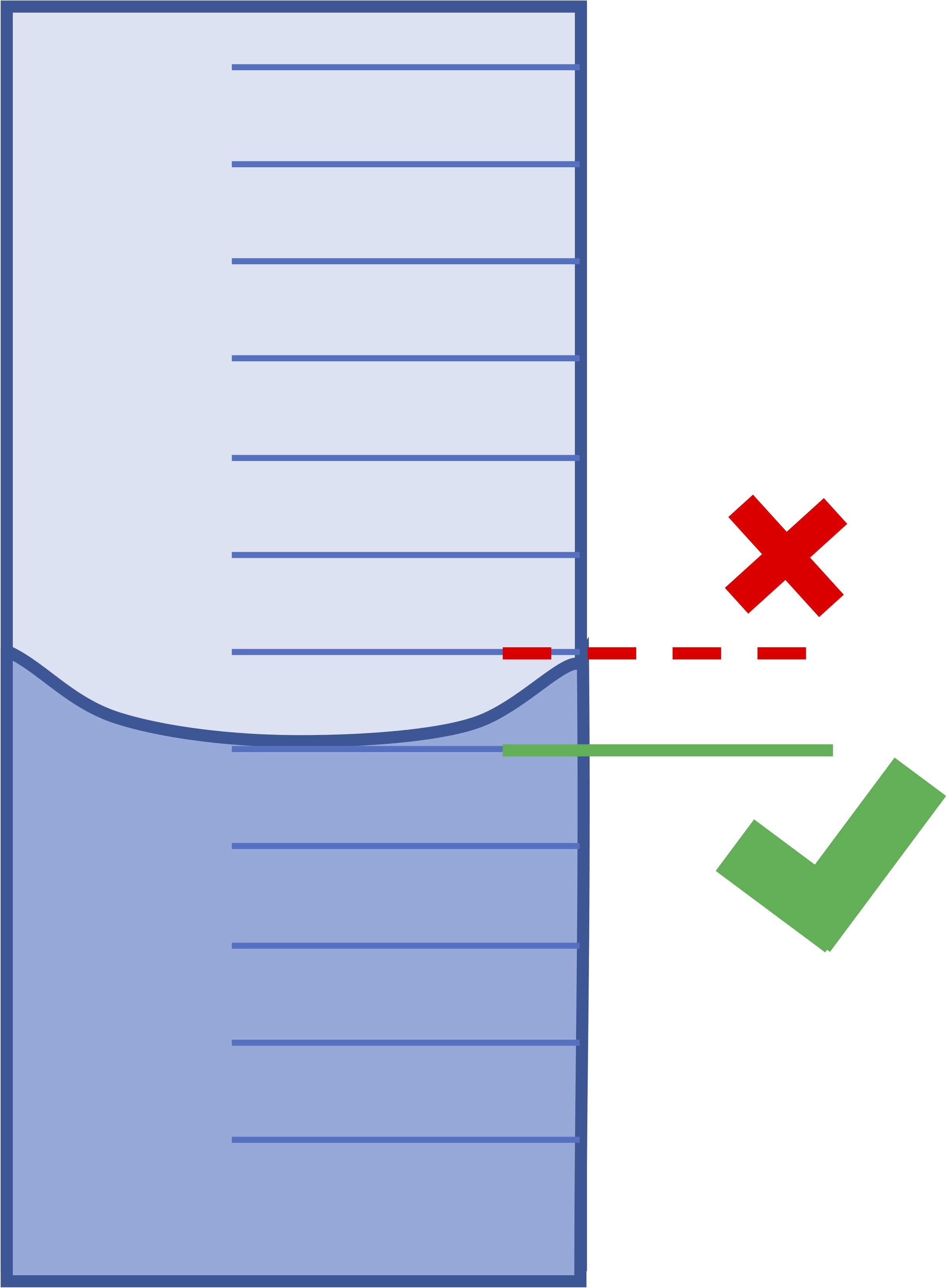## When Helping Means Winning contest

Ask a school-related question in the Help Zone and you could win one of three \$500 scholarships.

# Help Zone

### Student Question

Hi, I am having trouble reading a graduated cylinder. Could you help me?

Science## Explanations (1)

•Explanation from AlloprofExplanation from Alloprof

This Explanation was submitted by a member of the Alloprof team.

To read a graduated cylinder, there are 5 rules that you must absolutely respect. Here they are:

1. Choose the correct graduated cylinder size to increase accuracy. Always take the smallest possible graduated cylinder in which the entire volume of liquid to be measured fits. For example, if hesitating between a 5 mL graduated cylinder of 5 mL and a 10 mL graduated cylinder for a liquid volume of 4 mL, the 5 mL cylinder should be favoured because it is smaller than the other. By this method, measurement precision increases.

2. Calculate the value of the smallest gradation. To calculate the value of the smallest gradation, you must first choose two gradations whose volume measurement is written on the cylinder. For example, one could choose, in a 10 mL cylinder, the gradation of 4 mL and 5 mL (since gradations are spaced by 1 mL).As the space between 5 mL and 4 mL  contains 10 gradations, the formula is as follows:

$$smallest \; gradation = \frac{difference \; in \; volume}{number\; of\; small\; gradation}$$

$$smallest \; gradation = \frac{1\;mL}{10\;gradation}=0,1mL$$

3. Place the graduated cylinder on a flat and stable surface. This step is important to ensure a precise measurement.

4. Line up your eyes with the surface of the fluid. It is important, in order to counter the distortion caused by the glass of the cylinder, to position the eyes correctly toward the surface of the liquid in the graduated cylinder.

5. Read the volume while taking the meniscus into account (curved shape created on the walls of the container). Always measure volume from the bottom of the meniscus of the liquid.The green checkmark indicates where to read from (the bottom of the meniscus).

Don’t hesitate to ask other questions!